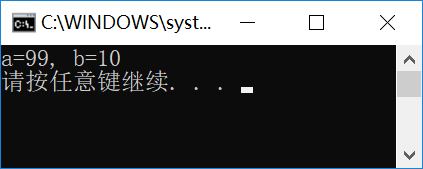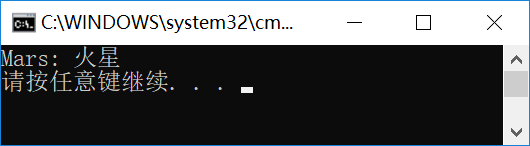## 泛型方法

``````using System;

namespace ConsoleTest
{
class Program
{
static void Main(string[] args)
{
int a = 10;
int b = 99;
Swap(ref a, ref b);
Console.WriteLine("a={0}, b={1}", a, b);
}
//
static void Swap(ref int x,ref int y)
{
int tmp = x;
x = y;
y = tmp;
}
}
}
````````````using System;

namespace ConsoleTest
{
class Program
{
static void Main(string[] args)
{
int a = 10;
int b = 99;
Swap(ref a, ref b);
Console.WriteLine("a={0}, b={1}", a, b);
}
//
static void Swap<T>(ref T x,ref T y)
{
T tmp = x;
x = y;
y = tmp;
}
}
}
``````

``````static T Test<T>(T x)
{
T y = x;
return y;
}
``````

## 泛型类

``````namespace ConsoleTest
{
public class CDictItem<K,V>
{
public K Key { get; set; }
public V Value { get; set; }
}
}
``````

``````static void Main(string[] args)
{
CDictItem<string, string> item = new CDictItem<string, string>();
item.Key = "Mars";
item.Value = "火星";
Console.WriteLine("{0}: {1}", item.Key, item.Value);
}
``````## 泛型接口

``````//
public interface  IG1<T>
{
T Data { get; set; }
void ShowData(T d);
}
//
public class CG1<T> : IG1<T>
{
public T Data { get; set; }
public void ShowData(T d)
{
Console.WriteLine(d == null ? "NULL" : d.ToString());
}
}
``````

## 泛型约束

``````public class CCls1<T> where T : I1
{
public T Obj { get; set; }
}
``````

CHY软件小屋原创作品！